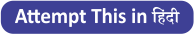Latest Banking jobs   »

# Quantitative Aptitude Quiz For RBI Assistant/ ESIC UDC Mains 2022- 14th April

Directions (1-5): Find the wrong number in the following number series questionsQ1. 12000 , 11880, 11520, 10920, 10080, 9120, 7680
(a) 7680
(b) 11520
(c) 9120
(d) 10080
(e) 10920

Q2. 18, 20 , 43, 133, 537, 2691, 16163
(a) 43
(b) 16163
(c) 133
(d) 537
(e) 2691

Q3. 180, 294, 458, 648, 900, 1210, 1584
(a) 180
(b) 1584
(c) 1210
(d) 294
(e) 458

Q4. 24, 48, 12, 96, 8, 192, 3
(a) 48
(b) 12
(c) 8
(d) 192
(e) 96

Q5. 1100, 1269, 1558, 1919, 2454, 3289, 4250
(a) 2454
(b) 1100
(c) 1919
(d) 3289
(e) 4250

Directions (6-10): What should come in place of question mark (?) in the following questions.

Q6. 25, 27, 33, ?, 65, 95
(a) 60
(b) 42
(c) 45
(d) 56
(e) 48

Q7. 560, 280, 700, 3150, 20475, ?
(a) 153562.5
(b) 174037.5
(c) 163800
(d) 194512.5
(e) 143325

Q8. 52, 46, 58, ?, 68, 26
(a) 38
(b) 40
(c) 36
(d) 42
(e) 32

Q9. 2, 6, 30, 210, 1890, ?
(a) 17790
(b) 21790
(c) 19790
(d) 18790
(e) 20790

Q10. 7500, 7379, 7210, 6921, 6560, ?
(a) 6076
(b) 5935
(c) 6031
(d) 6119
(e) 6160

Solutions

S1. Ans(c)
Sol.
Here, the pattern followed is,
12000 – (120 ×1)=11880
11880 – (120 ×3)=11520
11520 – (120 ×5)=10920
10920 – (120 ×7)=10080
10080 – (120 ×9)= 9000
9000 – (120 ×11)= 7680
So, wrong number is 9120 which should be replaced by 9000

S2. Ans(b)
Sol.
Here, the pattern followed is,
18 ×1 +2 =20
20 ×2 +3 =43
43 ×3 +4 =133
133 ×4 +5 =537
537 ×5 +6 =2691
2691 ×6 +7 =16153
So, wrong number is 16163 which should be replaced by 16153

S3. Ans(e)
Sol.
Here, the pattern followed is
(6)³ – (6)² =180
(7)³ – (7)² =294
(8)³ – (8)² =448
(9)³ – (9)² =648
(10)³ – (10)² =900
(11)³ – (11)² =1210
(12)³ – (12)² =1584
So, wrong number is 458 which should be replaced by 448

S4. Ans(c)
Sol.
24 × 2 = 48
48 ÷ 4 = 12
12 × 8 = 96
96 ÷ 16 = 6
6 × 32 = 192
192 ÷ 64 = 3
So, wrong number is 8 which should be replaced by 6

S5. Ans(a)
Sol.
Here, the pattern followed is
1100 + (13)² =1269
1269 + (17)² =1558
1558 + (19)² =1919
1919 + (23)² =2448
2448 + (29)² =3289
3289 + (31)² =4250
So, wrong number is 2454 which should be replaced by 2448

S6. Ans(c)
Sol.
Here, the pattern followed is,
25 + 1² + 1 = 27
27 + 2² + 2 = 33
33 + 3² + 3 = 45
45 + 4² + 4 = 65
65 + 5² + 5 = 95

S7. Ans(b)
Sol.
Here, the pattern followed is
560 × 0.5 = 280
280 × 2.5 = 700
700 × 4.5 = 3150
3150 × 6.5 = 20475
20475 × 8.5 = 174037.5

S8. Ans(a)
Sol.
Here, the pattern followed is
52-2×3=46
46+3×4=58
58-4×5=38
38+5×6=68
68-6×7=26

S9. Ans(e)
Sol.
Here, the pattern followed is
2×(1+2)=6
6×(2+3)=30
30×(3+4)=210
210×(4+5)=1890
1890×(5+6)=20790

S10. Ans(c)
Sol.
Here, the pattern followed is
7500-11²=7379
7379-13²=7210
7210-17²=6921
6921-19²=6560
6560-23²=6031#### Congratulations!Download Hindu Review of October 2021: Free PDF